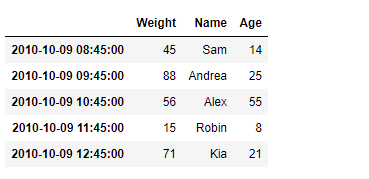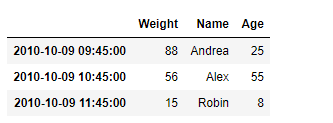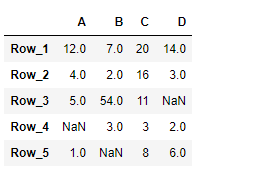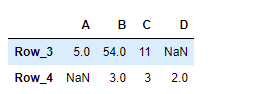Related Articles
Python | Pandas DataFrame.truncate
• Difficulty Level : Basic
• Last Updated : 21 Feb, 2019

Pandas DataFrame is a two-dimensional size-mutable, potentially heterogeneous tabular data structure with labeled axes (rows and columns). Arithmetic operations align on both row and column labels. It can be thought of as a dict-like container for Series objects. This is the primary data structure of the Pandas.

Pandas` DataFrame.truncate()` function is used to truncate a Series or DataFrame before and after some index value. This is a useful shorthand for boolean indexing based on index values above or below certain thresholds.

Syntax: DataFrame.truncate(before=None, after=None, axis=None, copy=True)

Parameter :
before : Truncate all rows before this index value.
after : Truncate all rows after this index value.
axis : Axis to truncate. Truncates the index (rows) by default.
copy : Return a copy of the truncated section.

Returns : The truncated Series or DataFrame.

Example #1: Use `DataFrame.truncate()` function to truncate some entries before and after the passed labels of the given dataframe.

 `# importing pandas as pd``import` `pandas as pd`` ` `# Creating the DataFrame``df ``=` `pd.DataFrame({``'Weight'``:[``45``, ``88``, ``56``, ``15``, ``71``],``                   ``'Name'``:[``'Sam'``, ``'Andrea'``, ``'Alex'``, ``'Robin'``, ``'Kia'``],``                   ``'Age'``:[``14``, ``25``, ``55``, ``8``, ``21``]})`` ` `# Create the index``index_ ``=` `pd.date_range(``'2010-10-09 08:45'``, periods ``=` `5``, freq ``=``'H'``)`` ` `# Set the index``df.index ``=` `index_`` ` `# Print the DataFrame``print``(df)`

Output :Now we will use `DataFrame.truncate()` function to truncate the entries before ‘2010-10-09 09:45:00’ and after ‘2010-10-09 11:45:00’ in the given dataframe.

 `# return the truncated dataframe``result ``=` `df.truncate(before ``=` `'2010-10-09 09:45:00'``, after ``=` `'2010-10-09 11:45:00'``)`` ` `# Print the result``print``(result)`

Output :As we can see in the output, the `DataFrame.truncate()` function has successfully truncated the entries before and after the passed labels in the given dataframe.

Example #2: Use `DataFrame.truncate()` function to truncate some entries before and after the passed labels of the given dataframe.

 `# importing pandas as pd``import` `pandas as pd`` ` `# Creating the DataFrame``df ``=` `pd.DataFrame({``"A"``:[``12``, ``4``, ``5``, ``None``, ``1``], ``                   ``"B"``:[``7``, ``2``, ``54``, ``3``, ``None``], ``                   ``"C"``:[``20``, ``16``, ``11``, ``3``, ``8``], ``                   ``"D"``:[``14``, ``3``, ``None``, ``2``, ``6``]}) `` ` `# Create the index``index_ ``=` `[``'Row_1'``, ``'Row_2'``, ``'Row_3'``, ``'Row_4'``, ``'Row_5'``]`` ` `# Set the index``df.index ``=` `index_`` ` `# Print the DataFrame``print``(df)`

Output :Now we will use `DataFrame.truncate()` function to truncate the entries before ‘Row_3’ and after ‘Row_4’ in the given dataframe.

 `# return the truncated dataframe``result ``=` `df.truncate(before ``=` `'Row_3'``, after ``=` `'Row_4'``)`` ` `# Print the result``print``(result)`

Output :As we can see in the output, the `DataFrame.truncate()` function has successfully truncated the entries before and after the passed labels in the given dataframe.

Attention geek! Strengthen your foundations with the Python Programming Foundation Course and learn the basics.

To begin with, your interview preparations Enhance your Data Structures concepts with the Python DS Course. And to begin with your Machine Learning Journey, join the Machine Learning – Basic Level Course

My Personal Notes arrow_drop_up